# Logistic Regression: A Detailed Overview

Logistic regression is one of the most used algorithms in industries these days. It is a supervised learning algorithm that predicts the categorical dependent variable (such as +/-; Type: A/B/C) using a given set of independent variables. This makes logistic regression a classification algorithm. Although it contains “regression” in its name, it solves the classification problem only, so please don’t be confused.

#### Key takeaways from this blog

After going through this blog, we will be able to answer:

1. What is a logistic regression model, and why is it a classification algorithm?
2. Why can we not directly fit a linear regression model on the same problem statement?
3. A detailed understanding of the Logit function and the cost function that makes this algorithm unique.
4. Hands-on for Logistic Regression.

Logistic Regression is like a linear regression algorithm, except it predicts categorical values instead of continuous data. This means that the true values (*y)* in logistic regression cannot be continuous like in linear regression. Instead, they are probability values between 0 and 1, classifying any observation into a particular category. In the image below, suppose there is a binary categorical target value which is probabilities. When we fit a linear regression model on this dataset, it will never be confined in the range of 0 and 1. But these are probabilities (let’s say p(X)), so how can we allow our model to go in the range of >1 and <0. That’s why we can not use linear regression here.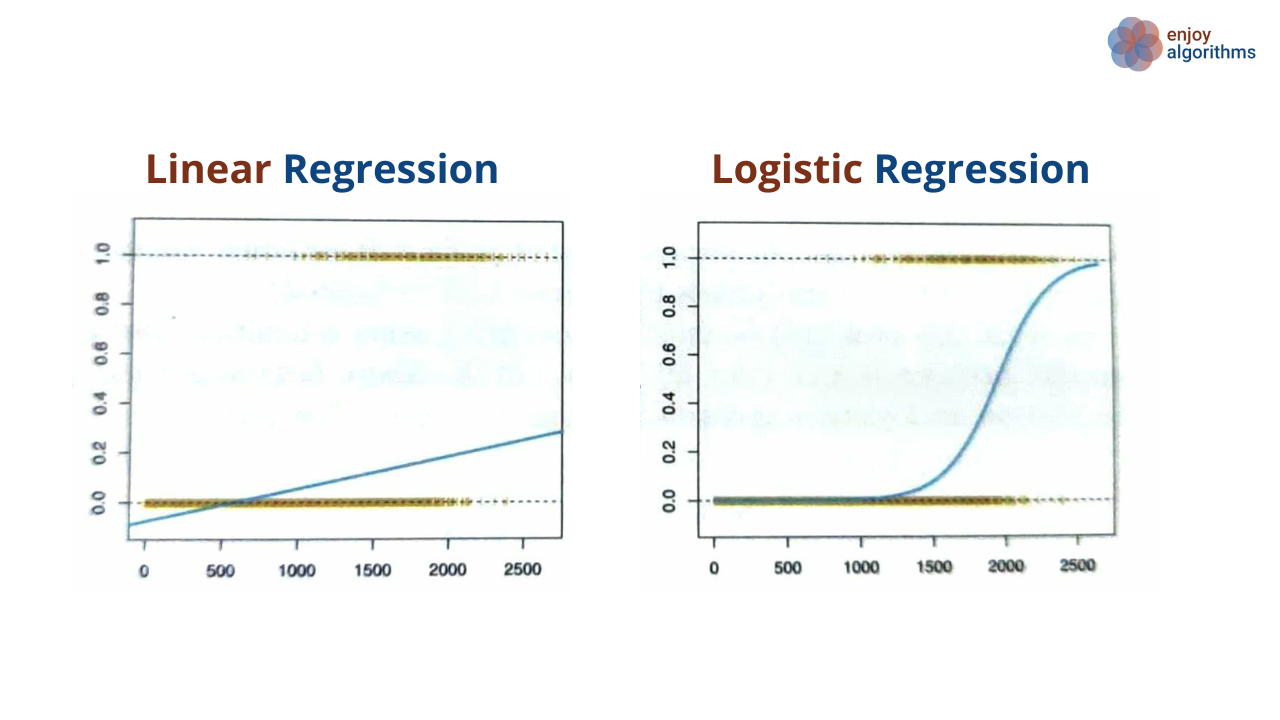Source: Introduction to statistical learning book

To avoid this situation, we must fit p(X) using some function that gives output always in the range of 0 and 1 for any value of X. There are many such functions, but in Logistic Regression, we use the logistic function.After arranging a little bit,Taking logarithm both the sides,Now, this is looking more like a Linear Regression problem where we can try to fit the logit function. The y-values (probability values) are transformed using the logit function (also known as a log of odds function) to make the problem more like a linear regression problem.If X = [x1, x2, …, xn], then we are trying to map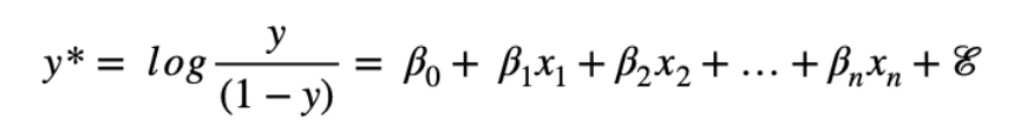We can say that the linear regression fits the linear function, but logistic regression fits the sigmoid function.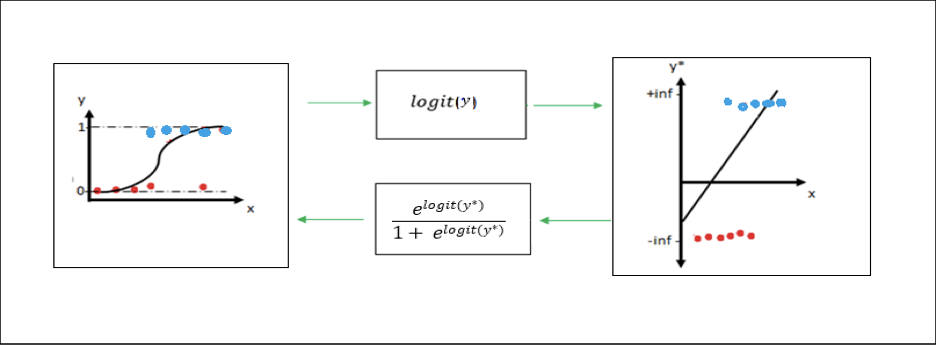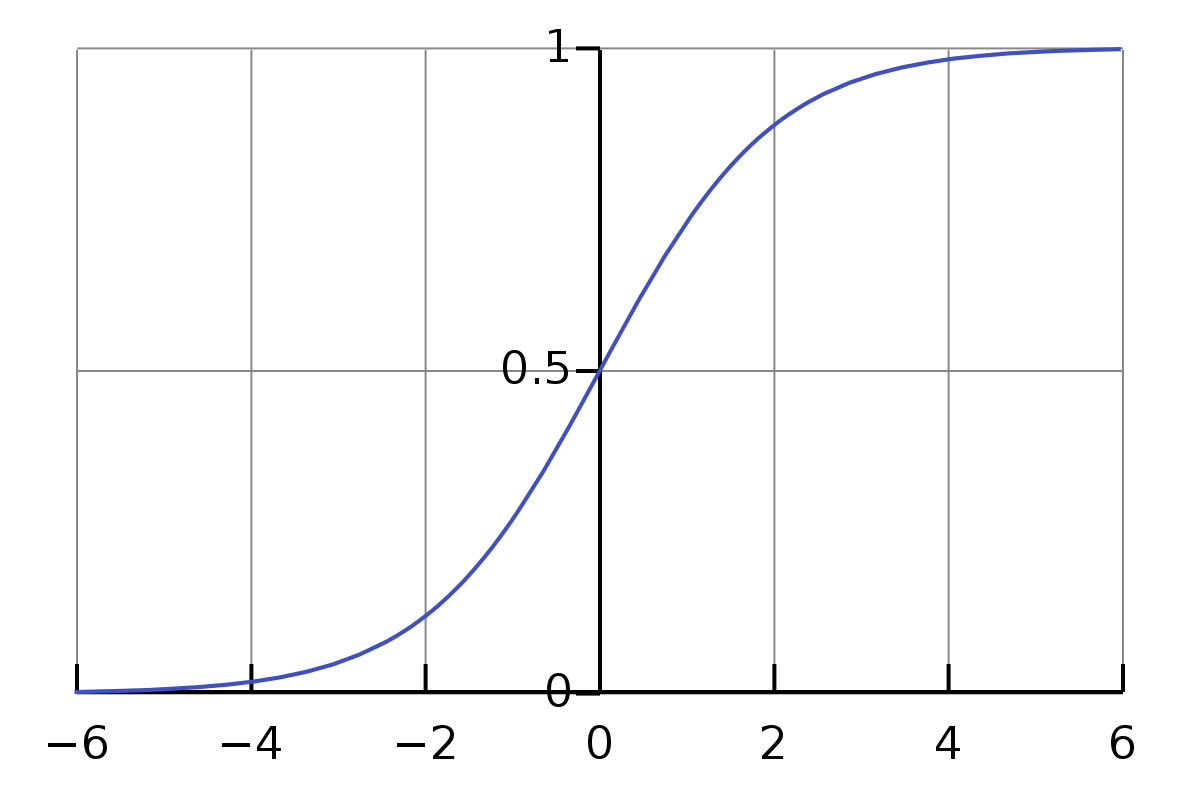Sigmoid function

#### Decision Boundary

We are saying that the logistic regression is mapping the categorical variables, and simultaneously we saw the equations that it predicts the sigmoid function, which is continuous. Isn’t it confusing?

Here comes the role of the decision boundary. Suppose our logistic regression model is trying to fit the categorical variables having values 0 and 1. We made our threshold be 0.5, which means, when the probability p(X) ≥ 0.5, it will be mapped to 1 otherwise 0. This “0.5” number can be different and depends upon the problem statement.

#### Loss Function

One significant difference between linear and logistic regression is that linear regression uses RMSE (Root Mean Squared Error) or Sum-Squared Error. In contrast, logistic regression cannot use the same, as the loss function will be non-convex, and primarily it will land in the local optima.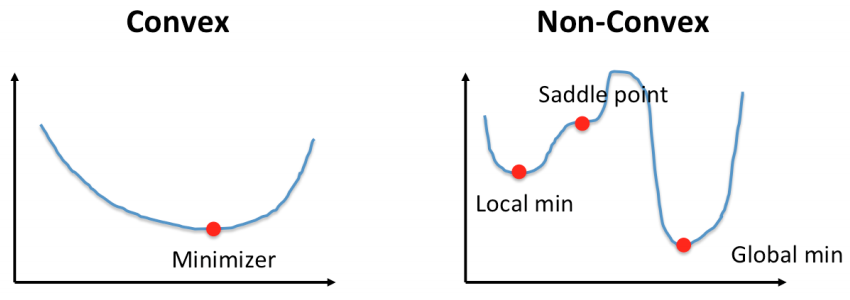Source: Pinterest

Therefore maximum-likelihood is to be adopted for this type of regression problem. In maximum likelihood, each point (in the (Y*-x) scale) is mapped to the initial line, and the values in the *y** scale correspond to the log(odds) values.The y values (in the y-X scale) can be computed using the equation above, and the likelihood of the y-values (or log-likelihood) can be calculated. The value y gives the probability of the observation having a positive class, and consecutively the negative class will have a probability of (1-y).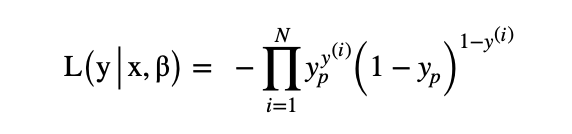The likelihood (or log(likelihood) is the cost function that is to be minimized, and that -ve sign in the above state makes sure of that. In simpler terms,If we take the logarithm on both sides,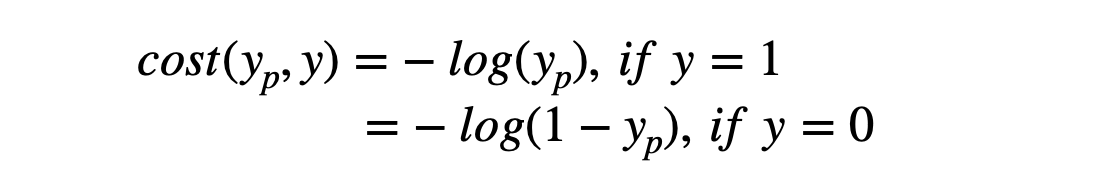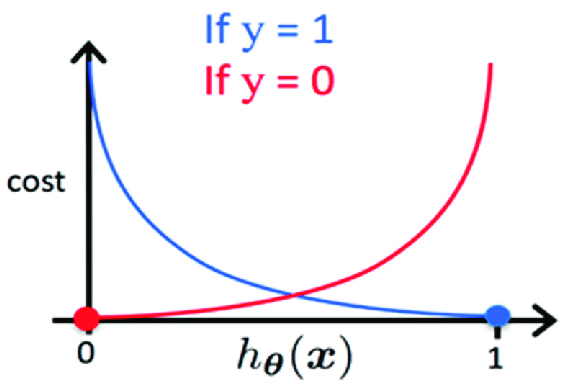Source: Researchgate

The loss function for the logistic regression algorithm is unique, and that's why we emphasized this section. R2 score for logistic regression is an appropriate estimate for determining the goodness of fit.

#### Types of Logistic Regression

Based on the nature of target variables, we can categorize logistic regression into three categories:

1. Binary/Binomial Logistic Regression: When the target variable can have the values in the binary format. E.g., (+ve and -ve), (email spam, non-spam), (black and white).
2. Multinomial Logistic Regression: The target variables can have >2 types of output classes but not in the ordered manner. E.g.,** (+ve, -ve, 0), *(black, white, gray)***
3. Ordinal Logistic Regression: The target variables can have >2 types of output classes but in an ordered manner. E.g., (Movie rating from 1 to 5).

Too much theory, let's move towards the implementation.

#### Python implementation of Logistic Regression

Step 1: The necessary libraries are imported.

• pandas for creating a data frame that is used to train and test the model.
• matplotlib for plotting scattered data points and fitted curves.
• traintestsplit for splitting the dataset into train and test sets.
• LogisticRegression from sklearn.linear_model for performing the classification operations
• Confusion_matrix from sklearn.metrics to evaluate the correctness of the model
``````import pandas as pd
import matplotlib.pyplot as plt
from sklearn.model_selection import train_test_split
from sklearn.linear_model import LogisticRegression
from sklearn.metrices import confusion_matrix
``````

The dataset used for this project is a college_admit dataset, which gives specific observations of students who were and weren’t admitted to a college based on their ‘sat’ score, ’gpa’, and the ‘number of recommendation’s they have. There are 55 observations and three features used to decide whether a student gets an admission or not.

``````path = 'college_admit.csv'
def data_set(path):
df = pd.DataFrame(data, columns= ['sat','gpa',
X = df[['sat','gpa', 'recommendations']]
print("DataFrame : ",df)
X_train,X_test,y_train,y_test = train_test_split(X,y, test_size=
0.2, random_state=0)
#It will split the data into train and test set in the ratio of
# 80:20
return (X_train,X_test,y_train,y_test)
``````

The data frame can be printed using the function data_set( ) above, which returns the training and testing dataset.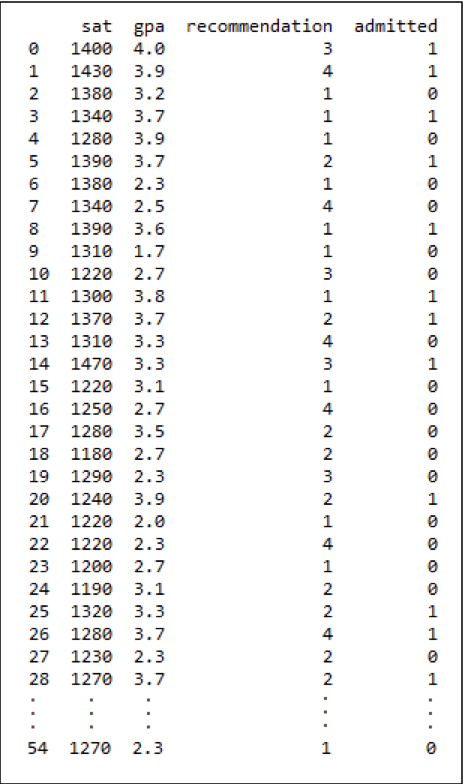Step 3: Training of Logistic Regression model

The model can be trained and returned using the function logistic_reg( ), which takes the output from the function data_set( ) as input, and returns a fully trained logistic regression model.

``````def logistic_reg(dataset):
X_train,X_test,y_train,y_test = dataset
model = LogisticRegression()
model.fit(X_train,y_train)
return model
``````

The function can return the model with its specifications.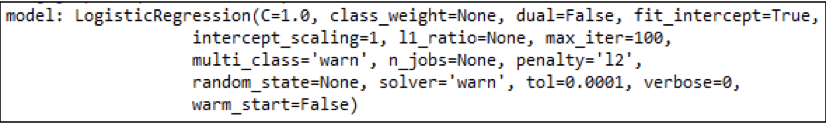Step 4: Evaluation of the trained model

Here we will compute and plot the confusion matrix to evaluate the classification performance. The confusion_matrix function is imported from sklearn.metrics library. It takes in the actual values of the test data (i.e., ytest) and the predicted values (i.e., ypred) by the model on the test data to give away a 2x2 confusion matrix.

``````if __name__ == "__main__":
dataset = data_set(path)
X_train,X_test,y_train,y_test = dataset
model = logistic_reg(dataset)
y_pred = model.predict(X_test)
confusion_mat = confusion_matrix(y_test,y_pred, labels=None)
print("Confusion Matrix = ",confusion_mat)
#ploting confusion matrix
fig,ax = plt.subplots()
ax.set_title("Confusion Matrix")
cm_ax = axx.matshow(confusion_mat)
fig.colorbar(cm_ax)
ax.set_xticklabels([''] + ['yes','no'])
ax.set_yticklabels([''] + ['yes','no'])
``````

The confusion matrix can be used to compute the model accuracy as –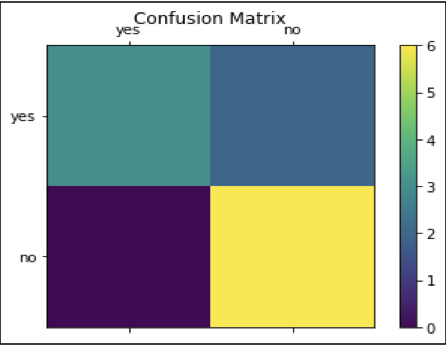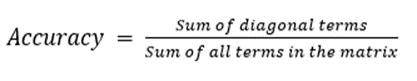Accuracy for our model is 9/11 = 0.8181

#### Quick Note

1. Logistic Regression can be used to predict the categorical dependent variable using a given set of independent variables.
2. Logistic regression is used for solving Classification problems, which means to predict the possibility of each observation.
3. The maximum likelihood estimation method is used as the objective function.
4. Logistic regression need not have any linear relationship between the dependent and independent variables.

#### Possible Interview Questions

Logistic regression is the most used classification algorithm and hence it is very popular in machine learning industries. Interviewers love to check the basic concepts around this algorithm. Some interview questions on this topic can be,

1. Why is Logistic Regression a classification problem?
2. Can we solve the classification problem using Linear Regression? If Yes, How? If No, what can be the technical challenges?
3. What are the types of Logistic Regression?
4. What is the cost function associated with Logistic Regression?
5. Why can’t we use MSE or other traditional cost functions instead of the log loss function?
6. What is the default value of the decision boundary? When do we need to change it?

#### Conclusion

In this blog, we represented a detailed understanding of Logistic Regression, one of the most used algorithms in industries. We learned about how this is different from the conventional linear regression algorithm. After that, we focused on the loss function/cost function for logistic regression, which makes it unique from other machine learning algorithms. After that, we did some hands-on Logistic regression and built a model to predict the probability of getting admission. We hope you have enjoyed the article.

#### Enjoy Learning! Enjoy Algorithms!

Get well-designed application and interview centirc content on ds-algorithms, machine learning, system design and oops. Content will be delivered weekly.